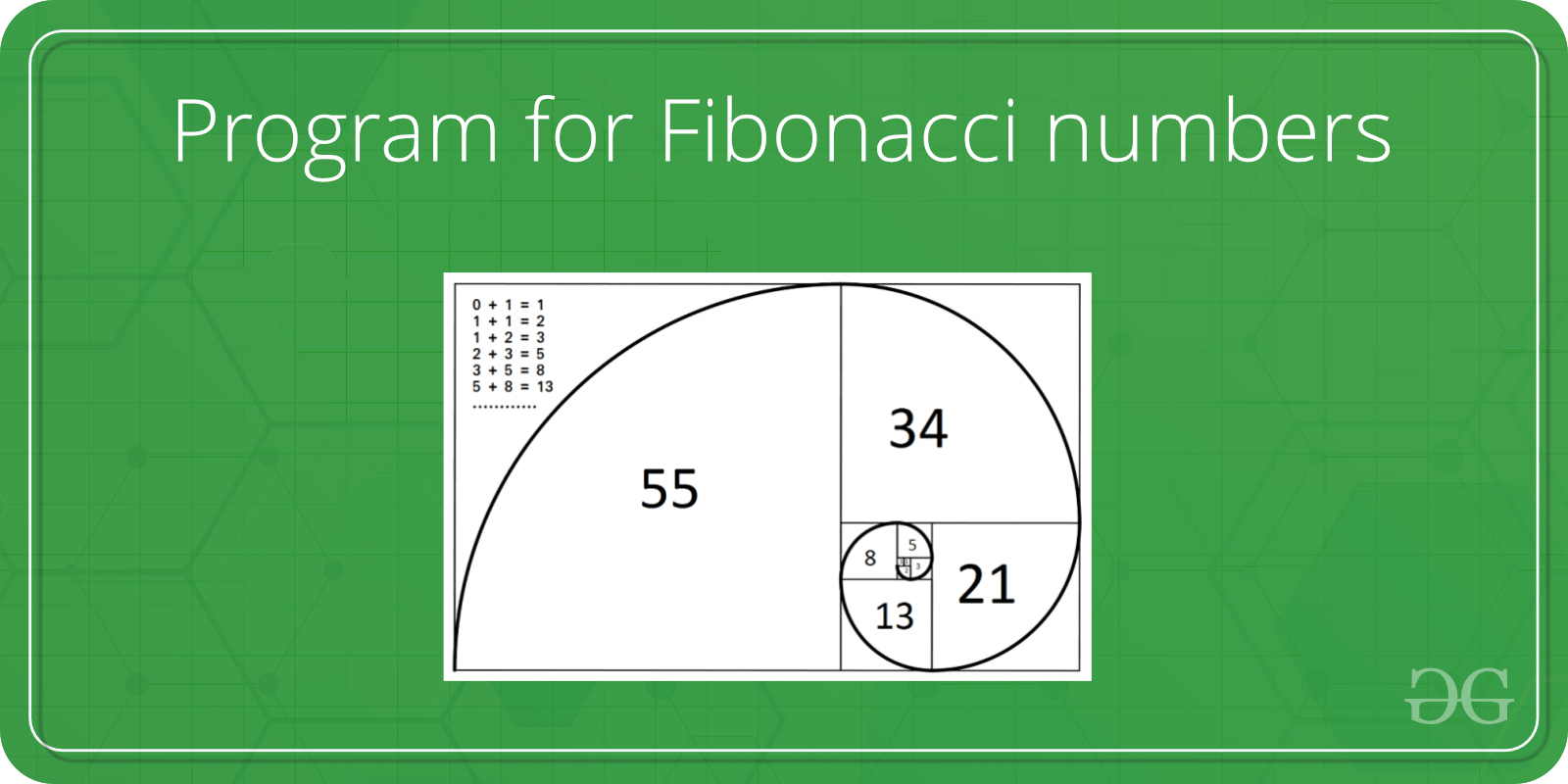Skip to content
Related Articles
TCS Coding Practice Question | Fibonacci Series
• Last Updated : 19 Sep, 2019

Given a number ‘n’, the task is to print the Fibonacci series using Command Line Arguments. The Fibonacci numbers are the numbers in the following integer sequence.

0, 1, 1, 2, 3, 5, 8, 13, 21, 34, 55, 89, 144, ……..

In mathematical terms, the sequence Fn of Fibonacci numbers is defined by the recurrence relation

`    Fn = Fn-1 + Fn-2`

with seed values

`   F0 = 0 and F1 = 1.`Examples:

```Input: n = 3
Output: 0, 1, 1

Input: 7
Output: 0, 1, 1, 2, 3, 5, 8
```

Approach:

• Since the number is entered as Command line Argument, there is no need for a dedicated input line
• Extract the input number from the command line argument
• This extracted number will be in String type.
• Convert this number into integer type and store it in a variable, say num
• Find the reverse of this number and store in a variable, say rev_num
• Check if this rev_num and num are same or not.
• If they are not same, the number is not Palindrome
• If they are same, the number is a Palindrome

Program:

## C

 `// C program to print the Fibonacci Series``// using command line arguments`` ` `#include ``#include /* atoi */`` ` `// Function to print the Fibonacci Series``void` `fib(``int` `n)``{`` ` `    ``int` `a = 0, b = 1, c, i;`` ` `    ``if` `(n <= 1)``        ``printf``(``"%d "``, a);``    ``else` `{`` ` `        ``printf``(``"%d %d "``, a, b);`` ` `        ``for` `(i = 3; i <= n; i++) {``            ``c = a + b;``            ``a = b;``            ``b = c;`` ` `            ``printf``(``"%d "``, c);``        ``}`` ` `        ``printf``(``"\n"``);``    ``}``}`` ` `// Driver code``int` `main(``int` `argc, ``char``* argv[])``{`` ` `    ``int` `num, res = 0;`` ` `    ``// Check if the length of args array is 1``    ``if` `(argc == 1)``        ``printf``(``"No command line arguments found.\n"``);`` ` `    ``else` `{`` ` `        ``// Get the command line argument and``        ``// Convert it from string type to integer type``        ``// using function "atoi( argument)"``        ``num = ``atoi``(argv);`` ` `        ``// Print the Fibonacci series``        ``fib(num);``    ``}``    ``return` `0;``}`

## Java

 `// Java program to print the Fibonacci Series``// using command line arguments`` ` `class` `GFG {`` ` `    ``// Function to print the Fibonacci Series``    ``static` `void` `fib(``int` `n)``    ``{`` ` `        ``int` `a = ``0``, b = ``1``, c, i;`` ` `        ``if` `(n <= ``1``)``            ``System.out.print(a + ``" "``);``        ``else` `{`` ` `            ``System.out.print(a + ``" "` `+ b + ``" "``);`` ` `            ``for` `(i = ``3``; i <= n; i++) {``                ``c = a + b;``                ``a = b;``                ``b = c;`` ` `                ``System.out.print(c + ``" "``);``            ``}`` ` `            ``System.out.println();``        ``}``    ``}`` ` `    ``// Driver code``    ``public` `static` `void` `main(String[] args)``    ``{`` ` `        ``// Check if length of args array is``        ``// greater than 0``        ``if` `(args.length > ``0``) {`` ` `            ``// Get the command line argument and``            ``// Convert it from string type to integer type``            ``int` `num = Integer.parseInt(args[``0``]);`` ` `            ``// Print the Fibonacci series``            ``fib(num);``        ``}``        ``else``            ``System.out.println(``"No command line "``                               ``+ ``"arguments found."``);``    ``}``}`

Output:
Want to learn from the best curated videos and practice problems, check out the C++ Foundation Course for Basic to Advanced C++ and C++ STL Course for the language and STL. To complete your preparation from learning a language to DS Algo and many more, please refer Complete Interview Preparation Course.

My Personal Notes arrow_drop_up